Get instant live expert help with Excel or Google Sheets“My Excelchat expert helped me in less than 20 minutes, saving me what would have been 5 hours of work!”

#### Post your problem and you’ll get expert help in seconds.

Your message must be at least 40 characters
Our professional experts are available now. Your privacy is guaranteed.

# Course completion status summaryRead time: 20 minutes

We use the COUNTIFS function and the IF function to develop a summary and display a course completion status based on a given set of data. The steps below will walk through the process.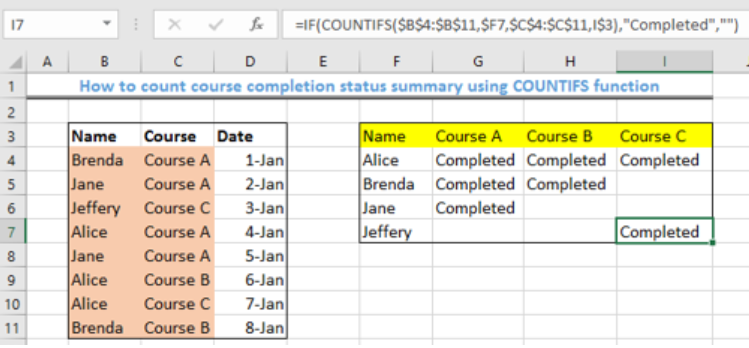Figure 1- Final Result of using the COUNTIFS Function

## General Formula

`=IF(COUNTIFS(range1, criteria1, range2, criteria2), [value_if_true], [value_if_false])`

## Formula

`=IF(COUNTIFS(\$B\$4:\$B\$11,\$F4,\$C\$4:\$C\$11,G\$3),"Completed","")`

## Setting up the Data

We will set up the table as shown in figure 2 to show the courses completed by some people. Each course is assumed to be completed by the individual in the same row as the course. Our objective is to track the number of courses completed by each participant.

• We will place the names of participants in range B4:B11
• The courses completed will be set in range C4:C11 and the date of completion will be entered in the range D4:D11.
• The array F3:I7 will contain the results.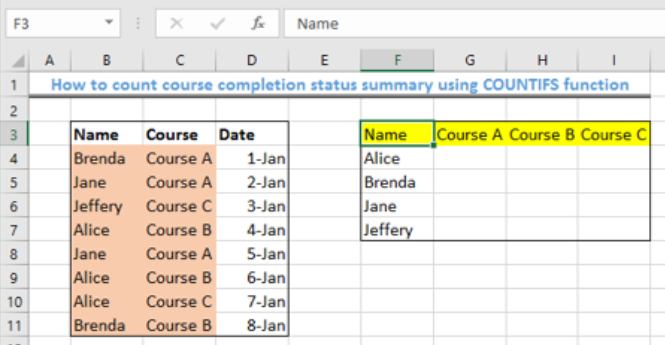Figure 2- Setting up the Data

## Applying the COUNTIFS and IF function

• We will click on Cell G4
• We will insert the formula below into Cell G4

`=IF(COUNTIFS(\$B\$4:\$B\$11,\$F4,\$C\$4:\$C\$11,G\$3),"Completed","")`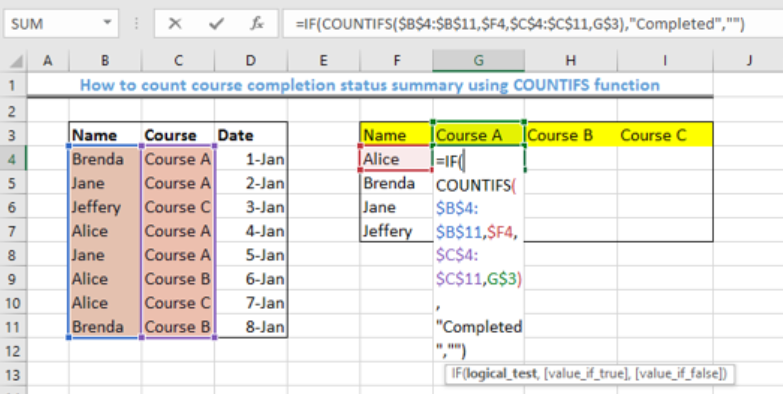Figure 3 – Use the COUNTIFS function for course completion status summary

• We will press the enter key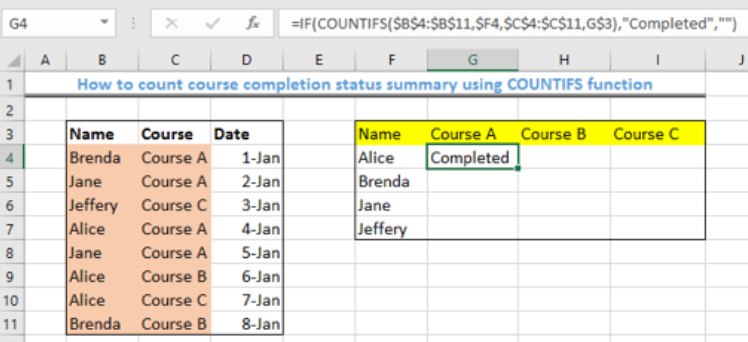Figure 4 – Applying the COUNTIFS function for course completion status summary

• We will use the fill handle (the small square box at the bottom right of Cell G4) to copy the formula to the other cells.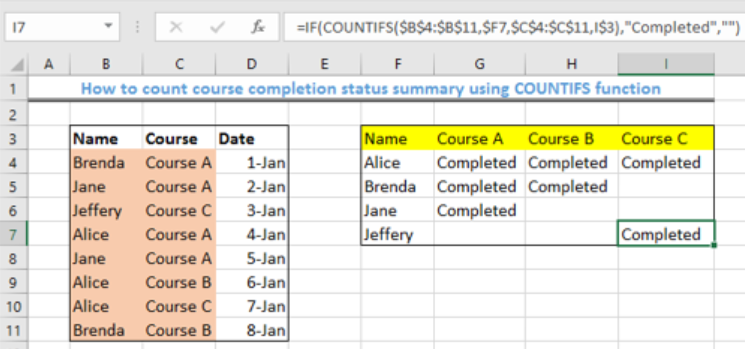Figure 5- Result of using the COUNTIFS and IF function

## Explanation

• COUNTIFS function

`= COUNTIFS(range1, criteria1, range2, criteria2)`

The COUNTIFS function matches the names of the participants with the criteria, which in this case is “completed.” When a name and a course is found and matched, the COUNTIFs function returns with the number 1, if they cannot be matched, then it returns with zero.

• IF function

`=IF(logical_test, [value_if_true], [value_if_false])`

`=IF(COUNTIFS(\$B\$4:\$B\$11,\$F4,\$C\$4:\$C\$11,G\$3),"Completed","")`

The IF Function evaluates the number, 1, returned by COUNTIFS as TRUE, which will be replaced by the term “completed”, and zero is FALSE, and therefore, the cell is left blank.

## Instant Connection to an Expert through our Excelchat Service

Most of the time, the problem you will need to solve will be more complex than a simple application of a formula or function. If you want to save hours of research and frustration, try our live Excelchat service! Our Excel Experts are available 24/7 to answer any Excel question you may have. We guarantee a connection within 30 seconds and a customized solution within 20 minutes.

### Did this post not answer your question? Get a solution from connecting with the expert.Another blog reader asked this question today on Excelchat:
Related blogs
Solution examplesCould you help me by explaining the IF function?
Solved by G. L. in 24 minsI have a question about Conditional Formatting. =IF('Sheet1'!A1="X",IF('Sheet1'!B1="Y", TRUE, FALSE),FALSE) the set the cell background to red. I would like to make it so that if Sheet1'!B1="Z" I set the back to blue. How can I achieve this.
Solved by B. F. in 39 minsI need to find out if the 2018 yearly sales goals were met if the yearly sales were \$25,000 or more using an IF logical function and to set the formula to return a value of YES if met, and NO if not
Solved by A. A. in 18 minsI filtered data in column A (it is labeled ID #) of a data set. On my next worksheet, column A is also the ID #, but it is a different data set. I want to filter the ID #'s the same for the two sheets, but how do I do that being that I am working with two different sets of data?
Solved by V. H. in 22 minsi want to create a very simple if function in a cell which is, if one cell is less than 1250, then the automatic value in the current cell is 250, dead easy, for someone who knows! Thank you.
Solved by A. H. in 14 mins## Subscribe to Excelchat.coAnother blog reader asked this question today on Excelchat: